Skip to content
Related Articles
ML | Matrix plots in Seaborn
• Last Updated : 26 Aug, 2019

Seaborn is a wonderful visualization library provided by python. It has several kinds of plots through which it provides the amazing visualization capabilities. Some of them include count plot, scatter plot, pair plots, regression plots, matrix plots and much more. This article deals with the matrix plots in seaborn.
Example 1: Heatmaps
Heatmap is a way to show some sort of matrix plot. To use a heatmap the data should be in a matrix form. By matrix we mean that the index name and the column name must match in some way so that the data that we fill inside the cells are relevant. Lets look at an example to understand this better.

Code : Python program

 `# import the necessary libraries``import` `seaborn as sns``import` `matplotlib.pyplot as plt ``%` `matplotlib inline `` ` `# load the tips dataset``dataset ``=` `sns.load_dataset(``'tips'``)`` ` `# first five entries of the tips dataset``dataset.head()`` ` `# correlation between the different parameters``tc ``=` `dataset.corr()`` ` `# plot a heatmap of the correlated data``sns.heatmap(tc)`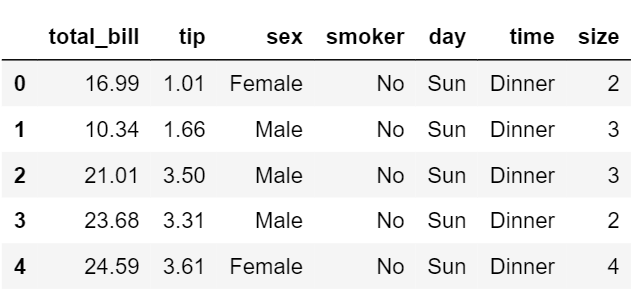The first five entries of the dataset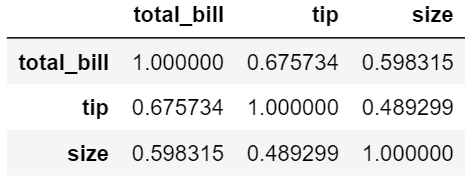The correlation matrix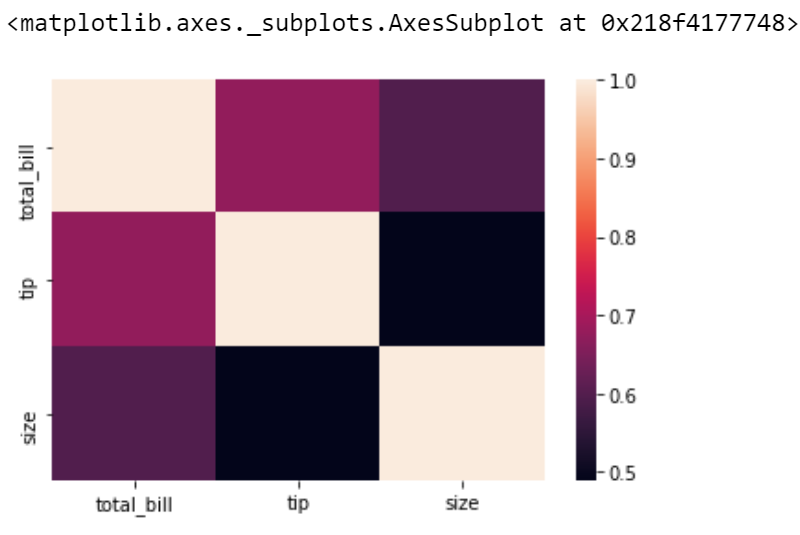Heatmap of the correlated matrix
Inorder to obatin a better visualisation with the heatmap, we can add the parameters such as annot, linewidth and line colour.

 `# import the necessary libraries``import` `seaborn as sns``import` `matplotlib.pyplot as plt ``%` `matplotlib inline`` ` `# load the tips dataset``dataset ``=` `sns.load_dataset(``'tips'``)`` ` `# first five entries of the tips dataset``dataset.head()`` ` `# correlation between the different parameters``tc ``=` `dataset.corr()``sns.heatmap(tc, annot ``=` `True``, cmap ``=``'plasma'``, ``            ``linecolor ``=``'black'``, linewidths ``=` `1``)`

Explanation

• annot is used to annotate the actual value that belongs to these cells
• cmap is used for the colour mapping you want like coolwarm, plasma, magma etc.
• linewidth is used to set the width of the lines separating the cells.
• linecolor is used to set the colour of the lines separating the cells.

Here is a plot that shows those attributes.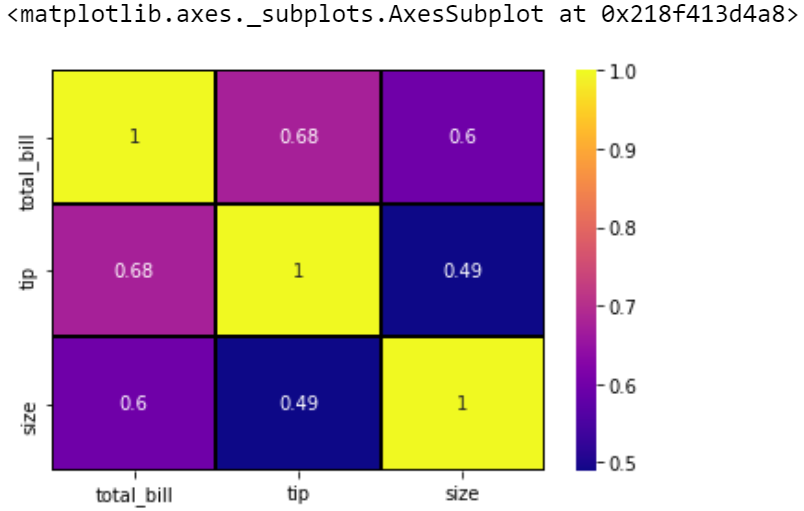So we can say that all a heatmap does is color the cells based on the gradient and uses some parameters to increase the data visualizaion.
Example 2: Cluster maps
Cluster maps use hierarchical clustering. It performs the clustering based on the similarity of the rows and columns.

 `# import the necessary libraries``import` `pandas as pd``import` `seaborn as sns``import` `matplotlib.pyplot as plt ``%` `matplotlib inline`` ` `# load the flights dataset``fd ``=` `sns.load_dataset(``'flights'``)`` ` `# make a dataframe of the data ``df ``=` `pd.pivot_table(values ``=``'passengers'``, index ``=``'month'``, ``                    ``columns ``=``'year'``, data ``=` `fd)`` ` `# first five entries of the dataset``df.head()`` ` `# make a clustermap from the dataset``sns.clustermap(df, cmap ``=``'plasma'``)`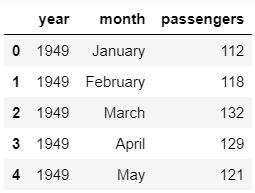The first five entries of the datasetThe matrix created using the pivot table(first five entries)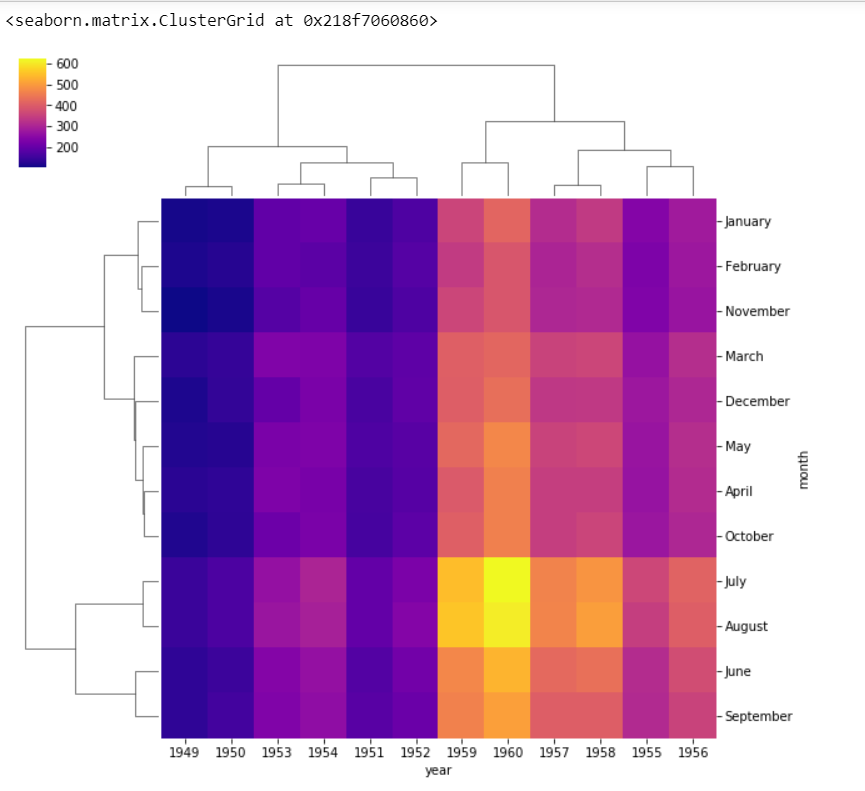Clustermap from the given data
We can also change the scale of the color bar by using the standard_scale parameter.

 `# import the necessary libraries``import` `pandas as pd``import` `seaborn as sns``import` `matplotlib.pyplot as plt ``%` `matplotlib inline`` ` `# load the flights dataset``fd ``=` `sns.load_dataset(``'flights'``)`` ` `# make a dataframe of the data ``df ``=` `pd.pivot_table(values ``=``'passengers'``, ``                    ``index ``=``'month'``, columns ``=``'year'``, data ``=` `fd)`` ` `# first five entries of the dataset``df.head()`` ` `# make a clustermap from the dataset``sns.clustermap(df, cmap ``=``'plasma'``, standard_scale ``=` `1``)`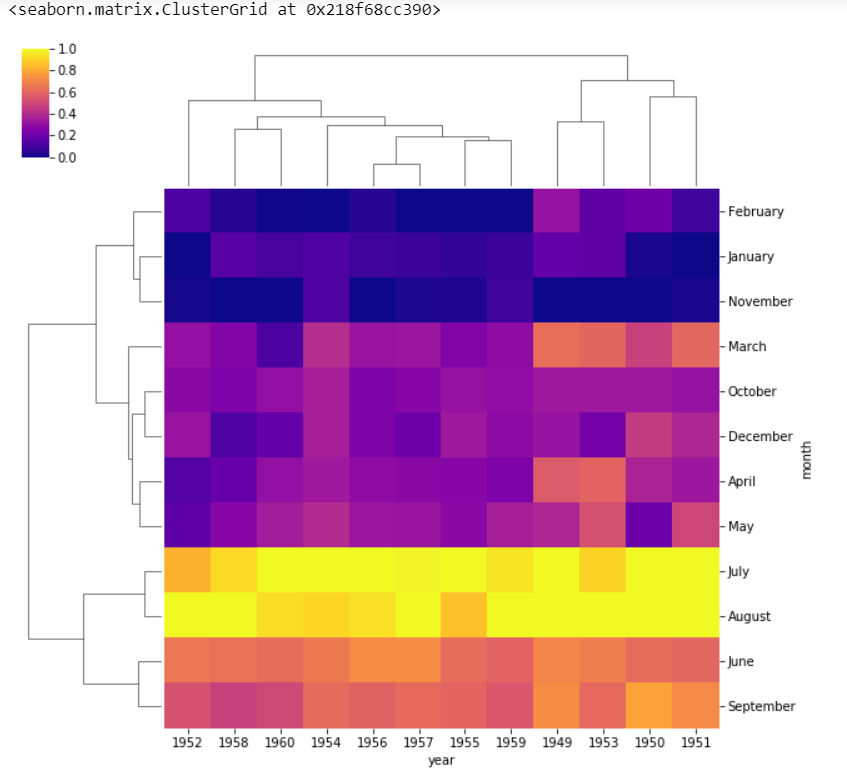Clustermap after using standard scaling
standard_scale = 1 normalises the data from 0 to 1 range. We can see that the months as well as years are no longer in order as they are clustered according to the similarity in case of clustermaps.
So we can conclude that a heatmap will display things in the order we give whereas the cluster map clusters the data based on similarity.My Personal Notes arrow_drop_up